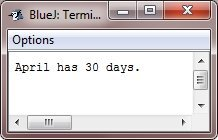# Array in Java with Examples

An array is a collection of variables of similar types that share a common name. Arrays of any type, with one dimension (single-dimensional arrays) or more (multidimensional arrays), can be created. The index of an array element is used to access it. Arrays are a convenient way to group related information.

## Types of Arrays in Java

There are two types of arrays in Java:

The "one-dimensional array" will be covered in this post immediately following this paragraph, while the "multidimensional array" will be covered in a separate (next) post.

## One-dimensional arrays in Java

A one-dimensional array is a data structure in Java that enables you to store a group of identically typed elements in a linear order. Another name for it is "single-dimensional array." Each element in the array can be accessed using an index starting from 0 to (length-1), where "length" is the number of elements in the array. The array has a fixed size that is specified when it is created.

To create an array, you first create an array variable of the desired data type. Here is the general form of a one-dimensional (1D) array declaration:

`type[] arrayName;`

"type" refers to the element type which is also called as the base type of the array and "arrayName" is the name of the array. For example:

`float[] marks;`

This statement declares an array called "marks" of the type float." You can define the size of the array using the "new" keyword to determine how many elements it can store. So if you want to go with the declaration of an array along with its size, then follow the following general form:

`type[] arrayName = new type[size];`

For example:

`float[] marks = new float;`

This statement declares an array named "marks" that can store up to ten elements, or numbers, of the "float" type.

Now, if you want to declare an array in Java with its initial values, then follow the following general form:

`type[] arrayName = {value1, value2, value3, ..., valueN};`

For example:

`float[] marks = {78.5, 98.9, 89}`

Here is another way to set up an array that has already been declared. Of course, you will use the "new" keyword.

```type[] arrayName;
arrayName = new type[]{value1, value2, value3, ..., valueN};```

For example:

```float[] marks;
marks = new float[]{78.5, 98.9, 89};```

### Java One-Dimensional Array Example

Putting together all the pieces of code, below is a program that creates an array of the number of days in each month:

```public class JavaProgram
{
public static void main(String args[])
{
int month_days[];
month_days = new int;

month_days = 31;
month_days = 28;
month_days = 31;
month_days = 30;
month_days = 31;
month_days = 30;
month_days = 31;
month_days = 31;
month_days = 30;
month_days = 31;
month_days = 30;
month_days = 31;

System.out.println("April has " + month_days + " days.");
}
}```

When the above Java program is compiled and executed, it will produce the following output:Java array indexes start from zero, so the number of days in April is month_days, or 30.

It is possible to combine the declaration of an array variable with the allocation of the array itself, as shown below.

`int month_days[] = new int;`

Here is another example that illustrates the concept and use of arrays in Java:

```public class JavaProgram
{
public static void main(String args[])
{
int[] arr = new int;
int i;

// initializing values for the array's elements
for(i=0; i<10; i++)
{
arr[i] = i;
}

// printing back all the values of the array
for(i=0; i<10; i++)
{
System.out.println("arr[" + i + "] = " + arr[i]);
}
}
}```

Here is the sample output of the above Java program:If there is an array, let's say

`Strings[] cities = {"Chicago", "Seattle", "New Orleans", "Boston"};`

Now if you want to change any element from the above array named cities," you can use its index number to access the position and initialize a new element to it. As an example, let's replace the city "Seattle" with Philadelphia."

```Strings[] cities = {"Chicago", "Seattle", "New Orleans", "Boston"};

Since indexing starts with 0, therefore, "cities" refers to the second element, not the first. Following is the complete example.

Java Code
```public class ReplaceCity {
public static void main(String[] args) {
String[] cities = {"Chicago", "Seattle", "New Orleans", "Boston"};

// print the original array
System.out.println("Original array: " + Arrays.toString(cities));

// print the updated array
System.out.println("Updated array: " + Arrays.toString(cities));
}
}
```
Output
```Original array: [Chicago, Seattle, New Orleans, Boston]
Updated array: [Chicago, Philadelphia, New Orleans, Boston]```

However, you can replace the following statement from the above Java program:

`cities = "Philadelphia";`

with the following block of code:

```for(int i = 0; i < cities.length; i++) {
if(cities[i].equals("Seattle")) {
break;
}
}```

Note: In the above program, the code "cities.length" returns the length of the "cities" array.

Java Online Test

« Previous Tutorial Next Tutorial »